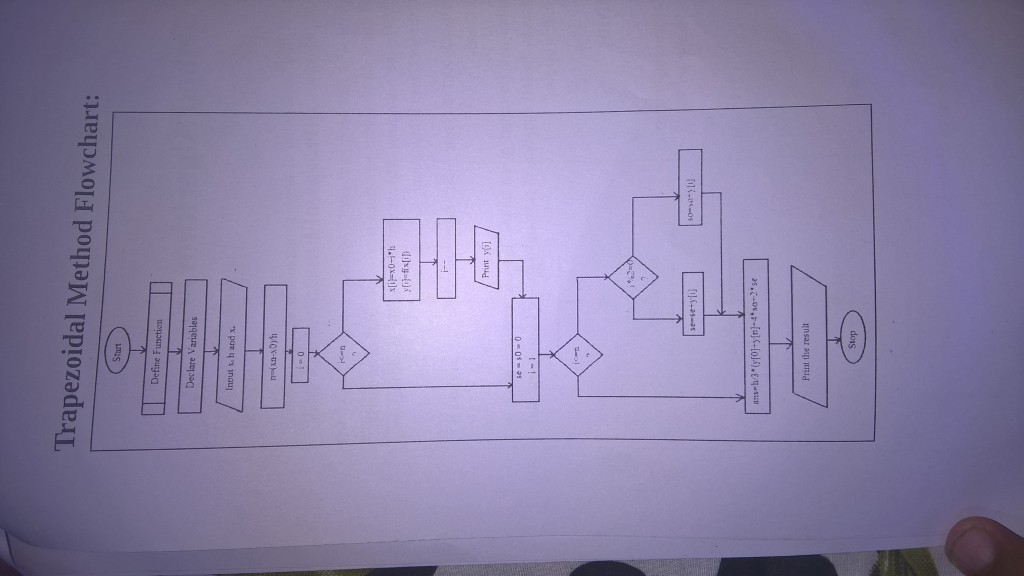# Trapezoidal Method Algorithm

• Start
• Define and Declare the function
• Input initial boundary value, final boundary value and length of interval
• Calculate number of strips, n=(final boundary value-initial boundary value)/length of interval
• Perform the following operations in loop
x[i]=x0+i*h
y[i]=f(x[i])print y[i]
• Initialize se=0,s0=0
• Do the following using a loop
if i%2=0
s0=s0+y[i]Otherwise
se=se+y[i]
• ans=h/3*(y+y[n]+4*s0+2*se)
• print the ans
• stop

Flow Chart:[wpedon id="7041" align="center"]